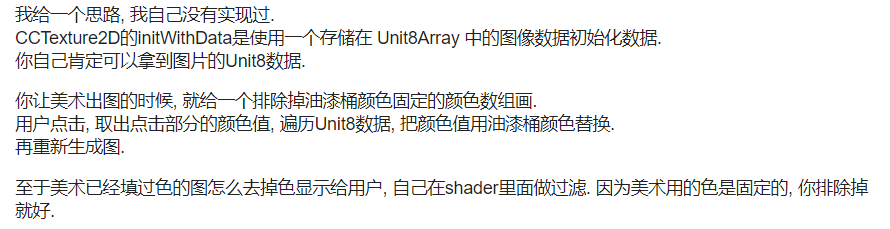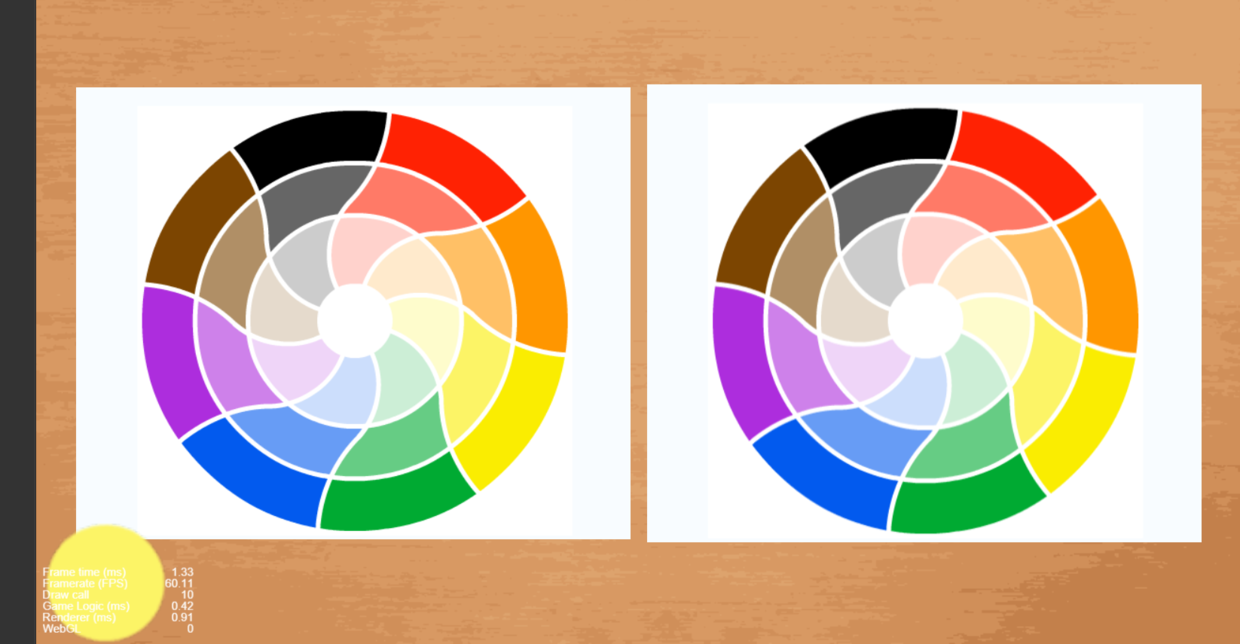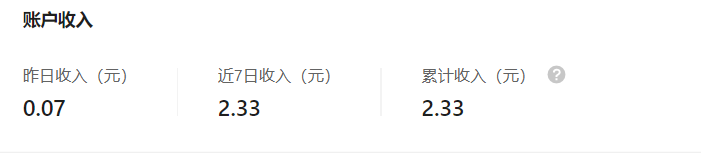# 前言# 制作方式

1. 先上一张图这是一张我在《绘图游戏调色盘取色方法》的文章中展示的一张图，图片左侧的色盘是原始图，右侧是使用相机截屏获得的数据更新后的图。当时是为了验证截屏的正确性而做的。这次正好用来做填色游戏。
2. 制作原理
实际上更改的就是右侧这张图使用的数据。左侧的图接收触摸事件，通过触摸点的位置，更新颜色数据。所以只要将两张图片重叠，用右边的图片盖住左边的图片，然后使用处理过的颜色数据更新右边的这张图，效果也就出来了。这里需要说一嘴，为什么要用两张图，就是相机截屏获得的数据初始化出来的纹理，正好是y反转的，所以右侧的图的scaleY我给了-1.否则就是倒着的。
3. 接收事件的处理
接收事件，获得点击坐标位置，这个没什么可多说的。
4. 更新数据
更新数据的时候我是从点击的点向四周扩散，因为颜色的点太多，所以不能用递归操作，因此做了每帧更新多少个点的方式，目前我给的是5000，OPPO A9真机实测不卡。使用一个数组记录更新过的点，避免重复更新消耗时间。
5. 坑与新玩法
我没有做在更新数据时不可以更换颜色操作，所以在更新数据时如果点击了左侧的色盘，那么右侧的颜色会跟着更新，这个也可以说是坑，也可以说是玩法。
色盘也是通过相机截屏获得的数据获取的，但是这种获取有缺陷，就是点击的色盘上的点不能有阴影，但是我使用的色盘刚好有，所以有的时候获取的颜色会是黑色或者灰色。

# 代码

import TextureRenderUtils from "../TextureRenderUtils";

const { ccclass, property } = cc._decorator;

@ccclass
export default class FillColorV1 extends cc.Component {

@property([cc.Component.EventHandler])
callback: cc.Component.EventHandler[] = [];

@property(cc.Camera)
camera: cc.Camera = null;

@property(cc.Sprite)
target: cc.Sprite = null;

@property(cc.Node)
renderNode: cc.Node = null;

private pointList: number[] = []
private r: number;
private g: number;
private b: number;

protected textureHelper: TextureRenderUtils = new TextureRenderUtils()

private grid: number[] = []

private imgData: ArrayBufferView = null;

start() {
this.node.on(cc.Node.EventType.TOUCH_START, this.touchStart, this)
this.init();
}

getTextureInfo() {
return this.textureHelper.getTextureInfo();
}

getDataUrl() {
return this.textureHelper.getDataUrl()
}

changeColor(color: cc.Color) {
this.r = color.r;
this.g = color.g;
this.b = color.b;

}

init() {

this.textureHelper.init(this.camera, this.renderNode)

this.textureHelper.render()

let data = this.textureHelper.getData()

if (data.length > 0) {
this.imgData = data;
cc.log('FillColorV1  width ', this.renderNode.width, ' height ', this.renderNode.height)
cc.log(' 实际上有多少个点  == ', data.length / 4)
let count = this.renderNode.width * this.renderNode.height;
cc.log(" 应该有多少个点的颜色 ", count)
if (this.target) {
let tTexture = this.target.spriteFrame.getTexture()
tTexture.setFlipY(false)
this.target.node.scaleY = -1

tTexture.initWithData(data, tTexture.getPixelFormat(), this.renderNode.width, this.renderNode.height)

}
}

}

update(dt: number) {
let flag = false;
let count = 0;
let width = this.textureHelper.width;
let r = this.r;
let g = this.g;
let b = this.b;
//当发现有点击坐标的时候开始执行。这个5000
while (this.pointList.length >= 2 && count++ <= 5000) {
flag = true;
let x = this.pointList.shift();
let y = this.pointList.shift();
this.paintPoint(x, y, width, r, g, b)
}

if (flag) {
let texture = this.target.spriteFrame.getTexture();
texture.initWithData(this.imgData, texture.getPixelFormat(), texture.width, texture.height)
}

}

paintPoint(x: number, y: number, width: number, r: number, g: number, b: number) {
let data = this.imgData;
let rowW = Math.floor(width) * 4//一行的长度
x = Math.floor(x)
let srow = Math.floor(y);//行开始位置
let startX = srow * rowW + x * 4;//列开始位置
if (!this.grid[startX]) {
this.grid[startX] = 1
// cc.log('r g b%{} ', data[startX + 0], data[startX + 1], data[startX + 2])
if (data[startX + 0] > 100 || data[startX + 1] > 100 || data[startX + 2] > 50) {
data[startX + 0] = r;
data[startX + 1] = g;
data[startX + 2] = b;

this.pointList.push(x - 1)
this.pointList.push(y);
this.pointList.push(x + 1)
this.pointList.push(y);
this.pointList.push(x)
this.pointList.push(y - 1)
this.pointList.push(x)
this.pointList.push(y + 1)
}
}
}
//用于打印点击的位置，无关紧要
showPointColor(x: number, y: number, width: number) {
let data = this.imgData;
let rowW = Math.floor(width) * 4//一行的长度
x = Math.floor(x)
let srow = Math.floor(y);//行开始位置
let startX = srow * rowW + x * 4;//列开始位置
cc.log('r g b', data[startX + 0], data[startX + 1], data[startX + 2])

}

touchStart(e: cc.Touch) {
let pos = e.getLocation();
pos = this.node.convertToNodeSpaceAR(pos)
cc.log('touchStart  x ', pos.x, ' y = ', pos.y)
this.pointList.length = 0;
this.grid.length = 0;
this.pointList.push(pos.x)
this.pointList.push(pos.y)
this.showPointColor(pos.x, pos.y, this.textureHelper.width)
}

}



# 结语# 欢迎扫码关注公众号《微笑游戏》，浏览更多内容。如果您觉得文章还可以，点赞、在看、分享、赞助、点下广告都是对我最大的鼓励，在下将感激不尽。posted @ 2020-08-02 10:49  游子陈  阅读(229)  评论(0编辑  收藏